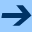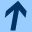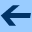The formal translation equation and formal cocycle equations for iteration groups of type I

## The formal translation equation and formal cocycle equations for iteration groups of type I

Jointly written with LUDWIG REICH.

Aequationes mathematicae 76, 54 - 91, 2008.

Abstract: We investigate the translation equation

(T) F(s+t,x)=F(s,F(t,x)),        s,t∈ ℂ,
and the cocycle equations
(Co1) α(s+t,x)= α(s,x)α(t,F (s,x)),        s,t∈ ℂ,
(Co2) β(s+t,x)= β(s,x)α(t,F (s,x)) +β(t,F (s,x)),         s,t∈ ℂ
in C[[x]], the ring of formal power series over ℂ. Here we restrict ourselves to iteration groups (F(s,x))s∈ ℂ of type I, i.e. to solutions of (T) of the form F(s,x)=c1(s)x+…, where c1 ≠ 1 is a generalized exponential function. It is easy to prove that the coefficient functions cn(s), αn(s), βn(s) of
 F(s,x)=.. ∑n≥ 1 cn(s)xn,    α(s,x)=.. ∑n≥ 0 αn(s)xn,    β(s,x)=.. ∑n≥ 0 βn(s)xn..
are polynomials in c1(s), polynomials or rational functions in one more generalized exponential function and, in a special case, polynomials in an additive function. For example, we obtain cn(s)=Pn(c1(s)), Pn(y)∈ ℂ[y], n≥ 1. For this we do not need any detailed information about the polynomials Pn.

Under some conditions on the exponential and additive functions it is possible to replace the exponential and additive functions by independent indeterminates. In this way we obtain formal versions of the translation equation and the cocycle equations in rings of the form (ℂ[y])[[x]], (ℂ[S,σ])[[x]], (ℂ(S))[[x]], (ℂ(S)[U])[[x]], and (ℂ(S)[σ,U])[[x]]. We solve these equations in a completely algebraic way, by deriving formal differential equations or Aczél-Jabotinsky type equations for these problems. It is possible to get the structure of the coefficients in great detail which are now polynomials or rational functions. We prove the universal character (depending on certain parameters) of these polynomials or rational functions. And we deduce the canonical form S-1(yS(x)) for iteration groups of type I. This approach seems to be more clear and more general than the original one. Some simple substitutions allow us to solve these problems in rings of the form (ℂ[[u]])[[x]], i. e. where the coefficient functions are formal series.

harald.fripertinger "at" uni-graz.at, October 10, 2019